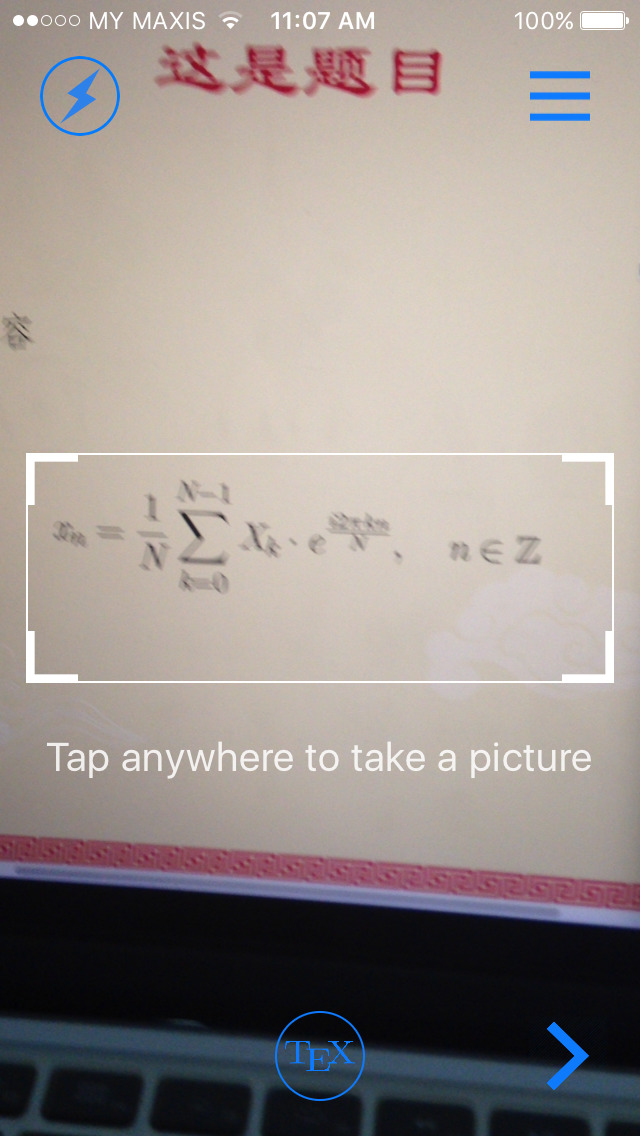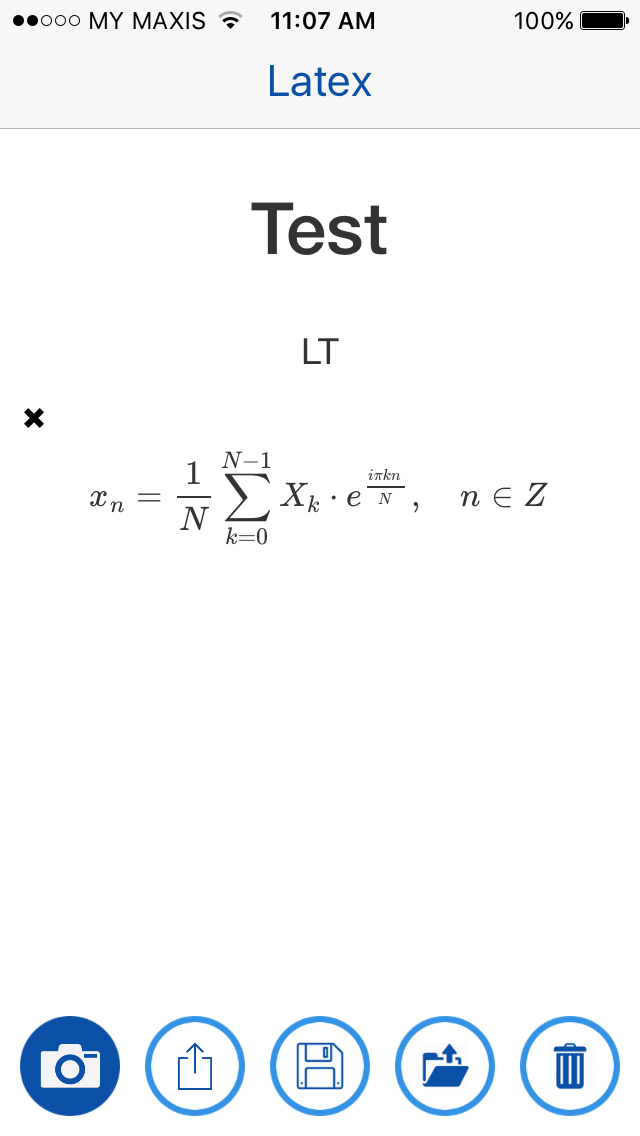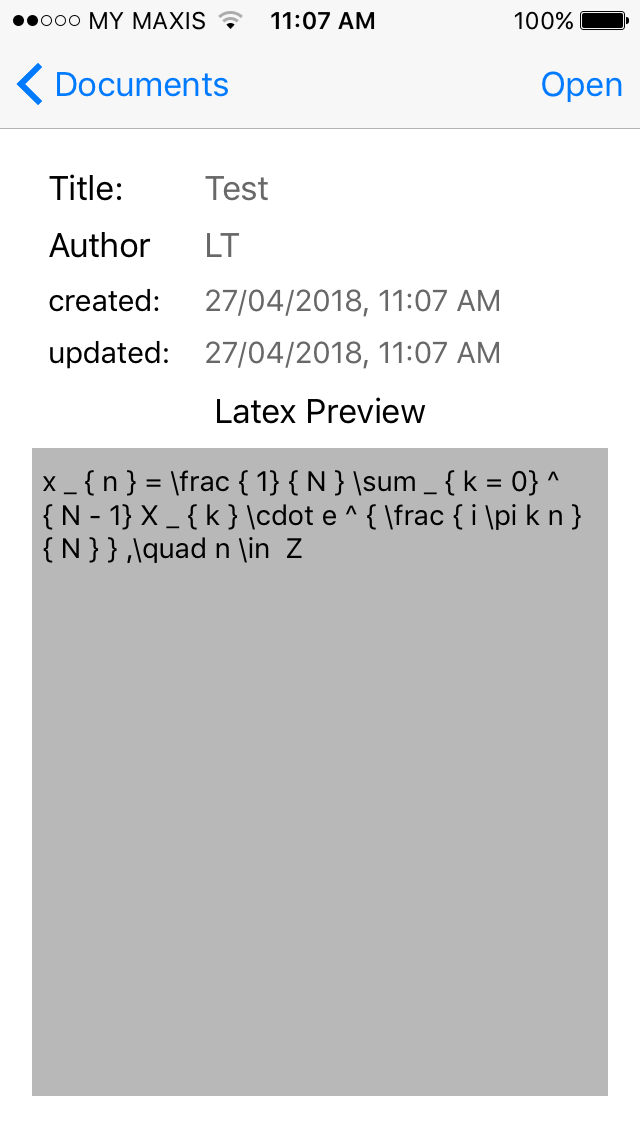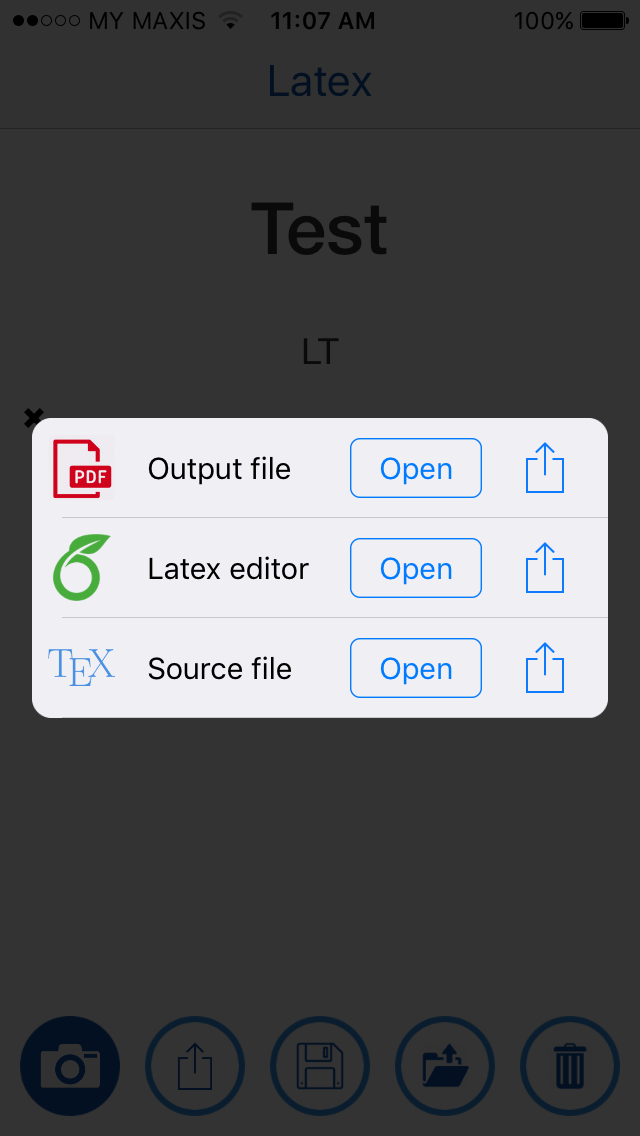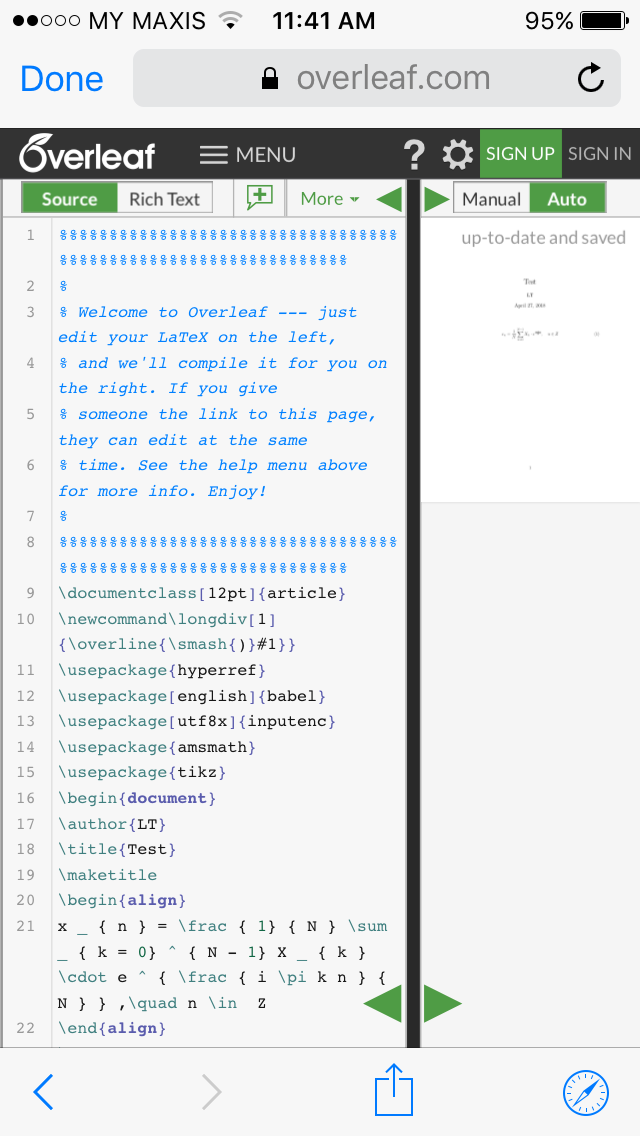Apart from online equation builders like CodeCogs or QuickMath which let you compose your mathematical formulae, there are other tools to help with quickly transcribing them to $$\mathrm{\LaTeX}$$.

MyScript Math lets you scribble mathematical formulae, then uses handwriting recognition to convert them into $$\mathrm{\LaTeX}$$ code (as well as MathML or export as an image).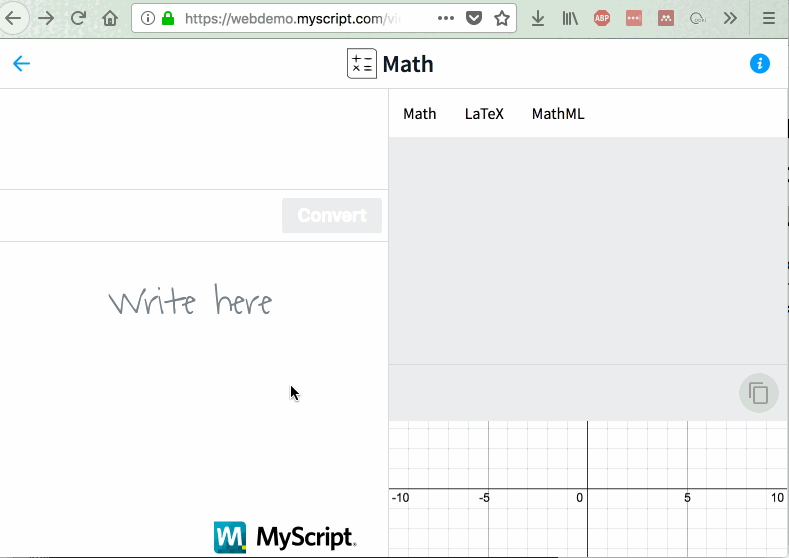MathPix can extract mathematical formulae from images. It is available as a MacOS app, which lets you "snip" formulae on your screen. Its Android and iOS apps lets you snap a picture of the formulae, and even has an option to open the converted $$\mathrm{\LaTeX}$$ code in an Overleaf project!